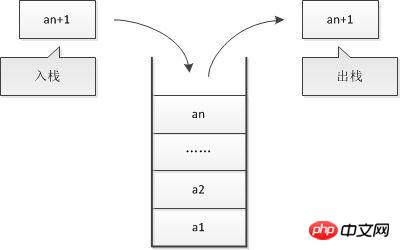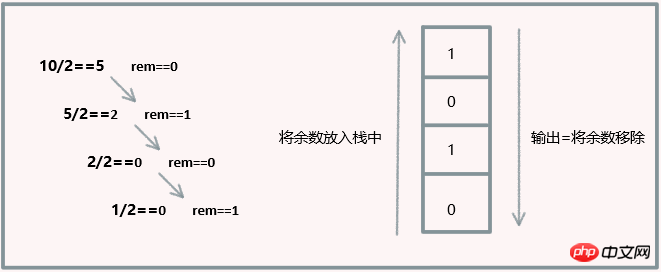• 注册

# 如何用JavaScript实现一个栈# 什么是栈（Stack）• 栈是一种遵从后进先出（LIFO）原则的有序集合。

• 新添加的或待删除的元素都保存在栈的末尾，称为栈顶，另一端叫栈底。

• 在栈里，新元素都靠近栈顶，旧元素都接近栈底

# 手动实现一个栈

`function Stack () { }`

```function Stack(){
var items = [];     //用来保存栈里的元素
}```

```push(element(s));   //添加新元素到栈顶
pop();              //移除栈顶的元素，同时返回被移除的元素
peek();             //返回栈顶的元素，不对栈做任何修改
isEmpty();          //如果栈里没有任何元素就返回true，否则false
clear();            //移除栈里的所有元素
size();             //返回栈里的元素个数,类似于数组的length属性```

```this.push = function (element) {
items.push(element);
}```

```this.pop = function () {
return items.pop();
}```

```this.peek = function () {
return items[items.length-1];
}```

```this.isEmpty = function () {
return items.length == 0;
}```

```this.size = function () {
return items.length;
}```

```this.clear = function () {
items = [];
}```

```this.print = function () {
console.log(items.toString());
}```

# 栈的完整代码

```function Stack(){

var items = [];     //用来保存栈里的元素

this.push = function (element) {
items.push(element);
}

this.pop = function () {
return items.pop();
}

this.peek = function () {
return items[items.length-1];
}

this.isEmpty = function () {
return items.length == 0;
}

this.size = function () {
return items.length;
}

this.clear = function () {
items = [];
}

this.print = function () {
console.log(items.toString());
}
}```

# 使用Stack类

```var stack = new Stack();
console.log(stack.isEmpty());         //控制台输出true```

```stack.push(5);
stack.push(8);```

`console.log(stack.peek());            //控制台输出8`

```stack.push(11);
console.log(stack.size());            //控制台输出3```

`stack.push(15);`

```stack.pop();
stack.pop();
console.log(stack.size());            //控制台输出2
stack.print();                        //控制台输出[5,8]```

# 用栈来解决问题```function pideBy2 (decNumber) {

var remStack = new Stack(),
rem,
binaryString = '';

while (decNumber>0) {  //{1}
rem = Math.floor(decNumber % 2);  //{2}
remStack.push(rem);  //{3}
decNumber = Math.floor(decNumber / 2);  //{4}
}

while (!remStack.isEmpty()) {  //{5}
binaryString += remStack.pop().toString();
}

return binaryString;
}```

```console.log(pideBy2(520));      //输出1000001000
console.log(pideBy2(10));       //输出1010
console.log(pideBy2(1000));     //输出1111101000```

```function baseConverter (decNumber, base) {

var remStack = new Stack(),
rem,
baseString = '';
digits = '0123456789ABCDEF';     //{6}

while (decNumber>0) {
rem = Math.floor(decNumber % base);
remStack.push(rem);  //{3}
decNumber = Math.floor(decNumber / base);
}

while (!remStack.isEmpty()) {
baseString += digits[remStack.pop()];  //{7}
}

return baseString;
}```

```console.log(baseConverter(1231,2));      //输出10011001111
console.log(baseConverter(1231,8));      //输出2317
console.log(baseConverter(1231,16));     //输出4CF```

# 小结• 相关标签：前端 数据结构与算法 javascript
• 本文原创发布php中文网 ，转载请注明出处，感谢您的尊重！
• 相关文章

• 原生javascript实现分享到朋友圈功能 支持ios和android_javascript技巧
• jQuery链式调用与show知识浅析_jquery
• JS实现登录页面记住密码和enter键登录方法推荐_javascript技巧
• BootstrapTable与KnockoutJS相结合实现增删改查功能【一】_javascript技巧
• 如何用JavaScript实现一个栈
• # 网友评论

文明上网理性发言，请遵守 新闻评论服务协议

我要评论

### 独孤九贱(5)_ThinkPHP5视频教程

ThinkPHP是国内最流行的中文PHP开发框架,也是您Web项目的最佳选择。《php.cn独孤九贱(5)－ThinkPHP5视频教程》课程以ThinkPHP5最新版本为例，从最基本的框架常识开始，将...

### 独孤九贱(4)_PHP视频教程

江湖传言：PHP是世界上最好的编程语言。真的是这样吗？这个梗究竟是从哪来的？学会本课程，你就会明白了。 PHP中文网出品的PHP入门系统教学视频，完全从初学者的角度出发，绝不玩虚的，一切以实用、有用...

### 独孤九贱(1)_HTML5视频教程

《php.cn原创html5视频教程》课程特色：php中文网原创幽默段子系列课程，以恶搞，段子为主题风格的php视频教程！轻松的教学风格，简短的教学模式，让同学们在不知不觉中，学会了HTML知识。 ...

### ThinkPHP5实战之[教学管理系统]

本套教程,以一个真实的学校教学管理系统为案例,手把手教会您如何在一张白纸上,从零开始,一步一步的用ThinkPHP5框架快速开发出一个商业项目。

### PHP入门视频教程之一周学会PHP

所有计算机语言的学习都要从基础开始，《PHP入门视频教程之一周学会PHP》不仅是PHP的基础部分更主要的是PHP语言的核心技术，是学习PHP必须掌握的内容，任何PHP项目的实现都离不开这部分的内容，通...

• 最新文章
• 热门排行